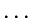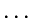Next: External Functions Up: Fitting of Data Previous: The Corrected Gauss-Newton No

# Function Specification

The functions to be fitted to data are linear combinations of a set of, so called, ``basic'' functions. Basic functions are either defined in the system or defined by the user as external FORTRAN routines. The actual combination of basic functions is defined via an interactive editor, (MIDAS command EDIT/FIT)

Basic functions are specified by the name, the independent variable(s) and parameter(s), with optional guesses for the parameters, following the syntax:

name(var1[,];par1[,]) [par1=value]The function name name defines the basic function used, it can be a system function, as defined in the table 8.1, or a external function with name USER00, ..., USER09. In this case, the corresponding file(s) USER00.FOR, ..., USER09.FOR will exist in the working area and will contain the definition of the routines following the syntax described in the next section.

The number of independent variables of the function is determined by the string var1[,]. The actual names of the independent variables are considered as dummy names but their number has to coincide with the actual number of parameters of the function. All the functions defined in a given fit must have the same number of independent variables.

Parameters are defined by unique names after the semicolon in the function specification. Parameters are interpreted according to the position and to the number of independent variables in the function.

A parameter is generally given a first guess on the same line, as pari=value, it can also be fixed to a given value or kept proportional to another parameter. The parameter is defined as fixed with the symbol @ immediately following the value as pari=value@. Linear constrains between parameters are defined as pari=parj*value or pari=parj/value.

According to these rules, a one dimensional gaussian function is specified with the EDIT/FIT command as

1 GAUSS(X;A,B,C) A=10. B=3200.@ C=1.
where X is the dummy name of the only independent variable, the first parameter, defining the maximum of the function, is called A, initialized to 10, the second parameter, defining the position of the gaussian, is called B, and its fixed value is 3200 in world coordinates, and the FWHM is the parameter C, with initial value 1.

A linear combination of a gaussian and a Cauchy distribution, centered at the same position is specified as

1 GAUSS(X;A1,B1,C1) A1=10. B1=3200. C1=1.
2 CAUCHY(X;A2,B2,C2) A2=A1/10. B2=B1 C2=4.
in this case, the maximum of the Cauchy distribution is determined by the corresponding parameter of the Gaussian.

We include in table 8.1 a summary of the system basic functions; the actual mathematical expressions, with the meaning of the function parameters are given in section 8.8.

Table 8.1: Basic Fit Functions
 POLY(X;A,B,...) polynomial (1D, 2D) LOG(X;A,B,C) natural logarithm EXP(X;A,B,C) exponential SIN(X;A,B,C) sinus TAN(X;A,B,C) tangent SINC(X;A,B,C) sinc SINCS(X;A,B,C) sinc square GAUSS(X;A,B,C) (FWHM) Gaussian distribution (1D) GAUSS(X,Y;A,B,C,D,E,F) (FWHM) Gaussian distribution (2D) GAUSSA(X;A,B,C) (Standard) Gaussian distribution (1D) CAUCHY(X;A,B,C) Cauchy distribution (1D) CAUCHY(X,Y;A,B,C,D,E,F) Cauchy distribution (2D) LORENTZ(X,Y;A,B,C,D,E,F) Modified Cauchy (Lorentz) distribution LAPLACE(X;A,B,C) Laplace distribution TRIANG(X;A,B,C) Triangular distribution POISSON(X;A,B,C) Poisson distribution IGAUSS(X;A,B,C) Integrated (FWHM) Gaussian distribution (1D) IGAUSSA(X;A,B,C) Integrated (Standard) Gaussian distribution (1D)Next: External Functions Up: Fitting of Data Previous: The Corrected Gauss-Newton No
http://www.eso.org/midas/midas-support.html
1999-06-09